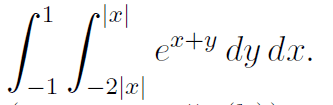# Double integral absolute value.

## Homework Statement

I just need to know if what i did is correct. The question is as follows:

http://imgur.com/1RL7e## The Attempt at a Solution

What I did is as follows:

I split this integral into two parts and solved.

[integral from 0 to 1 [integral from x to -2x (e^(x+y)) dy] dx] +
[integral from -1 to 0 [integral from -x to 2x (e^(x+y)) dy] dx]

The way of thinking about it is that y goes from x to -2x when x goes from 0 to 1 and -x to 2x when x goes from -1 to 0.

Is that right?